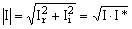# Reminder on complex numbers

## Form of a complex number

A complex number I can be written in different forms:

• in Cartesian form:

I = Ir + j Ii

where:

Ir : real part of I

Ii : imaginary part of I

j: pure imaginary number with j² = –1

• in Polar form (or exponential form):

where:

I mod : modulus of I

ϕ : argument of I (or phase)

j: pure imaginary number with j² = –1

## Conjugate of a complex number

The conjugate of I is defined by:

I* = Ir – j Ii

## Modulus of a complex number

The modulus of I is defined by: